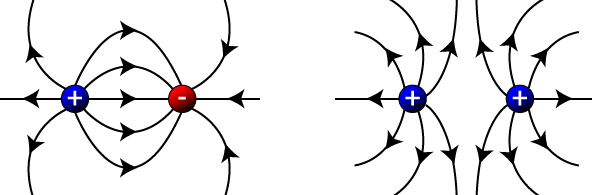# Electric Fields

Also similar to gravity, the electrostatic force is a non-contact force. Charged objects do not have to be in contact with each other to exert a force on each other. Somehow, a charged object feels the effect of another charged object through space. The property of space that allows a charged object to feel a force is a concept called electric field. Although we cannot see an electric field, we can detect its presence by placing a positive test charge at various points in space and measuring the force the test charge feels.

While looking at gravity, the gravitational field strength was the amount of force obsered by a mass per unit mass. The electric field strength is the amount of electrostatic force observed by a charge per unit charge. Therefore, the electric field strength, E, is the electrostatic force observed at a given point in space divided by the test charge itself. Electric field strength is measured in Newtons per Coulomb (N/C).Question: Two oppositely charged parallel metal plates, 1.00 centimeter apart, exert a force with a magnitude of 3.60×10–15 newtons on an electron placed between the plates. Calculate the magnitude of the electric field strength between the plates.

Answer:Question: Which quantity and unit are correctly paired?

1. resistivity and Ω/m
2. potential difference and eV
3. current and C•s
4. electric field strength and N/C

Answer: (4) electric field strength and N/C.

### Electric Field Lines

Since we can't actually see the electric field, we can draw electric field lines to help us visualize the force a charge would feel if placed at a specific position in space. To help us visualize the electric field, we can draw electric field lines in space. These lines show the direction a positive charged particle would feel a force if it were placed at that point in space. The more dense the lines are, the stronger the force a charged particle would feel, therefore the stronger the electric field. As the lines get further apart, the strength of the electric force a charged particle would feel is smaller, therefore the electric field is smaller.

By convention, we draw electric field lines showing the direction of force on a positive charge. Therefore, to draw electric field lines for a system of charges, follow these basic rules:

1. Electric field lines point away from positive charges, and toward negative charges.
2. Electric field lines never cross.
3. Electric field lines always intersect conductors at right angles to the surface.
4. Stronger fields have closer lines.
5. Field strength and line density decreases as you move away from the charges.

Let's take a look at a few examples of electric field lines, starting with isolated positive (left) and negative (right) charges. Notice that for each charge, the lines radiate outward or inward spherically. The lines point away from the positive charge, since a positive test charge placed in the field (near the fixed charge) would feel a repelling force. The lines point in toward the negative fixed charge, since a positive test charge would feel an attractive force.If you have both positive and negative charges in close proximity, you follow the same basic procedure:Of course, electric field lines actually lie in three dimensions, as demonstrated in this video animation.

Courtesy Penn State Schuylkill

### Comparing Electrostatics & Gravity

Because gravity and electrostatics have so many similarities, let's take a minute to do a quick comparison of electrostatics and gravity.

Electrostatics

Force:Field Strength:Field Strength:Electrostatic Constant:Charge Units: Coulombs

Gravity

Force:Field Strength:Field Strength:Gravitational Constant:Mass Units: kilogramsThe big difference between electrostatics and gravity? The gravitational force can only attract, while the electrostatic force can both attract and repel. Notice again that both the electric field strength and the gravitational field strength follow the inverse-square law relationship. Field strength is inversely related to the square of the distance.